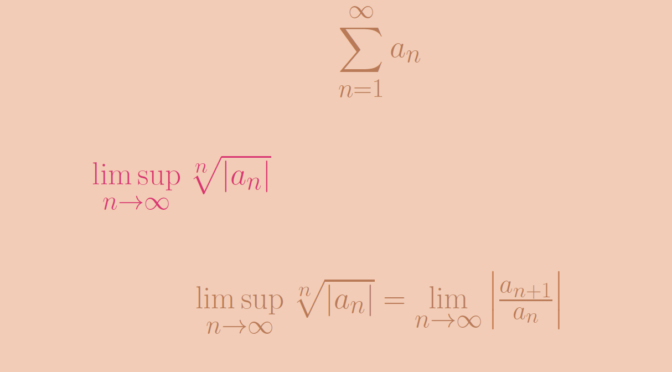# Root test

The root test is a test for the convergence of a series $\sum_{n=1}^\infty a_n$ where each term is a real or complex number. The root test was developed first by Augustin-Louis Cauchy.

We denote $l = \limsup\limits_{n \to \infty} \sqrt[n]{\vert a_n \vert}.$ $$l$$ is a non-negative real number or is possibly equal to $$\infty$$. The root test states that:

• if $$l < 1$$ then the series converges absolutely;
• if $$l > 1$$ then the series diverges.

The root test is inconclusive when $$l = 1$$.

### A case where $$l=1$$ and the series diverges

The harmonic series $$\displaystyle \sum_{n=1}^\infty \frac{1}{n}$$ is divergent. However $\sqrt[n]{\frac{1}{n}} = \frac{1}{n^{\frac{1}{n}}}=e^{- \frac{1}{n} \ln n}$ and $$\limsup\limits_{n \to \infty} \sqrt[n]{\frac{1}{n}} = 1$$ as $$\lim\limits_{n \to \infty} \frac{\ln n}{n} = 0$$.

### A case where $$l=1$$ and the series converges

Consider the series $$\displaystyle \sum_{n=1}^\infty \frac{1}{n^2}$$. We have $\sqrt[n]{\frac{1}{n^2}} = \frac{1}{n^{\frac{2}{n}}}=e^{- \frac{2}{n} \ln n}$ Therefore $$\limsup\limits_{n \to \infty} \sqrt[n]{\frac{1}{n^2}} = 1$$, while the series $$\displaystyle \sum_{n=1}^\infty \frac{1}{n^2}$$ is convergent as we have seen in the ratio test article.

### Comparison of the ratio test and the root test

One can prove that if $$\lim\limits_{n \to \infty} \left\vert \frac{a_{n+1}}{a_n} \right\vert$$ exists, the equality $\limsup\limits_{n \to \infty} \sqrt[n]{\vert a_n \vert} = \lim\limits_{n \to \infty} \left\vert \frac{a_{n+1}}{a_n} \right\vert$ holds. Hence if the ratio test is conclusive, so is the root test. However the root test can succeed while the ratio test fails as shown by following example:
$a_n=\begin{cases} \frac{1}{2^n} & \text{if } n \text{ is odd}\\ \frac{1}{2^{n-2}} & \text{if } n \text{ is even} \end{cases}$ for which $$\limsup\limits_{n \to \infty} \sqrt[n]{\vert a_n \vert}=\frac{1}{2}$$ while
$\frac{a_{n+1}}{a_n}=\begin{cases} 2 & \text{if } n \text{ is odd}\\ \frac{1}{8} & \text{if } n \text{ is even} \end{cases}$ In that case the root test allows to conclude that the series converges and the ratio test is inconclusive as $$\lim\limits_{n \to \infty} \left\vert \frac{a_{n+1}}{a_n} \right\vert$$ doesn’t exist.